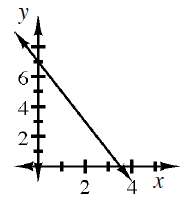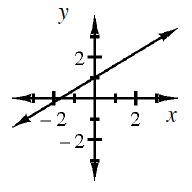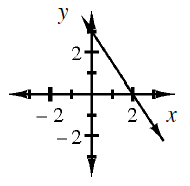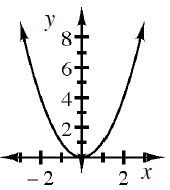### Home > INT3 > Chapter 1 > Lesson 1.1.1 > Problem1-7

1-7.

In this course, you will learn strategies that allow you to make sketches of many different types of graphs. However, when the directions tell you to make a complete graph, this means you must do more than make a quick sketch. To make a complete graph, you need to:

• Use Graph Paper.

• Plot Points Accurately.

• Scale and label axes.

• Label Key Points.

On separate sets of axes, make a complete graph of each of the following functions.

1. $y = -2x + 7$

Graph the $y$-intercept first. $(0,7)$

Notice the NEGATIVE slope.1. $y=\frac { 3 } { 5 }x+1$1. $3x + 2y = 6$

Solve for $y$ before graphing.

$y=-\frac{3}{2}x+3$1. $y = x^2$

Try making a table. Remember to use negative numbers.